学习SVM（四） 理解SVM中的支持向量（Support Vector）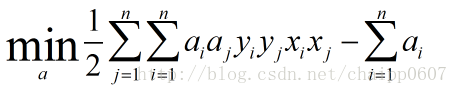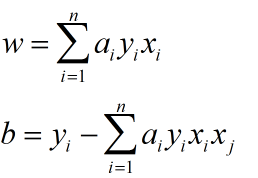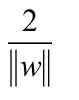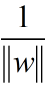，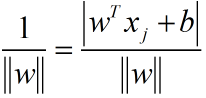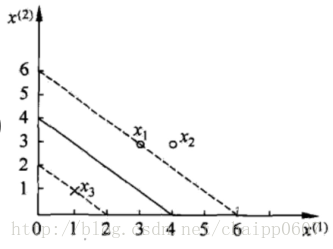a1*a1，a2*a2，a3*a3，2*a1*a3，2*a1*a2，2*a2*a3，举个例子确定前面的系数：
C*a1*a2 = [(x1)(x2)*y1*y2]a1*a2
C=2*[(3,3)(4,3)](1)(1)=2(12+9)=42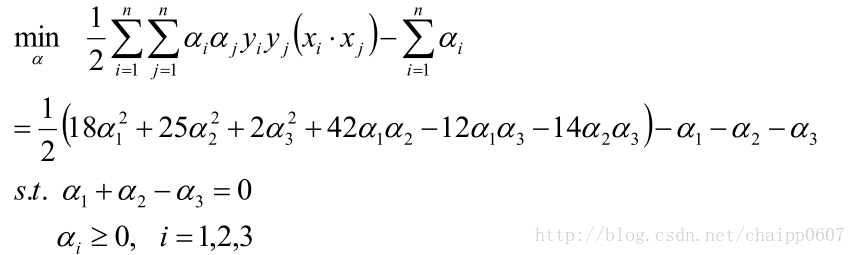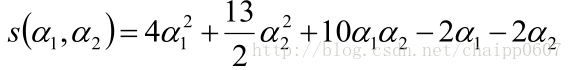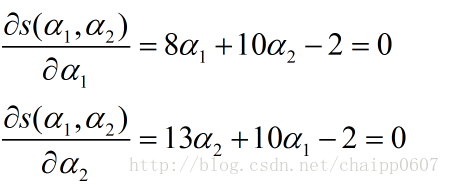a1 = 1.5
a2 = -1

a1=0时，我们应该把a1的值往s对a2的偏导里面带入：
a2=2/13 （满足条件）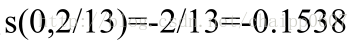a2=0时，我们应该把a2的值往s对a1的偏导里面带入：
a1=1/4 （满足条件）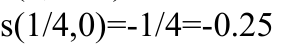a1 = 1/4
a2 = 0
a3 = 1/4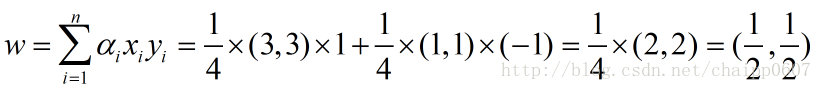w1=w2=0.5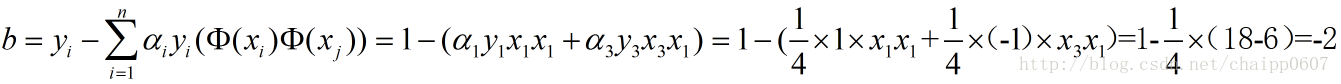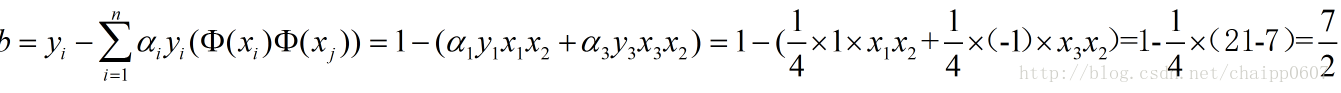0.5x1+0.5x2+2=0